Most Affordable JEE | NEET | 8,9,10 Preparation by Kota's Top IITian Doctor Faculties

# Electrochemistry - JEE Main Previous Year Questions with SolutionsJEE Main Previous Year Papers Questions of Chemistry with Solutions are available at eSaral. Practicing JEE Main chapter wise questions of Chemistry will help the JEE aspirants in realizing the question pattern as well as help in analyzing weak & strong areas.   Simulator   Previous Years AIEEE/JEE Mains Questions
Q. Given : $\mathrm{E}_{\mathrm{Fe}^{-3} / \mathrm{Fe}}^{\circ}=-0.036 \mathrm{V}, \quad \mathrm{E}_{\mathrm{Fe}^{-2} / \mathrm{Fe}}^{\circ}=-0.439 \mathrm{V}$. The value of standard electrode potential for the change. $\mathrm{Fe}^{+3}_{(\mathrm{aq})}+\mathrm{e}^{-} \longrightarrow \mathrm{Fe}^{+2}_{(\mathrm{aq})}$ will be :- (1) 0.770 V (2) –0.27 V (3) –0.072 V (4) 0.385 V AIEEE 2009
Ans. (1) $\mathrm{E}^{\circ}=\frac{3 \times-0.036+2 \times 0.439}{1}=0.77 \Rightarrow \mathrm{A}$
Q. The Gibbs energy for the decomposition of $\mathrm{Al}_{2} \mathrm{O}_{3}$ at $500^{\circ} \mathrm{C}$ is as follows : $\frac{2}{3} \mathrm{Al}_{2} \mathrm{O}_{3} \longrightarrow \frac{4}{3} \mathrm{Al}+\mathrm{O}_{2}, \mathrm{AG}=+966 \mathrm{KJ} \mathrm{mol}^{-1}$ The potential difference needed for electrolytic reduction of $\mathrm{Al}_{2} \mathrm{O}_{3}$ at $500^{\circ} \mathrm{C}$ is at least :- (1) 5.0 V      (2) 4.5 V       (3) 3.0 V         (4) 2.5 V AIEEE 2010
Ans. (4) $966 \times 10^{3}=4 \times 96500 \times \mathrm{E} \Rightarrow \mathrm{E}=2.5 \mathrm{V}$
Q. Resistance of 0.2 M solution of an electrolyte is $50 \Omega$. The specific conductance of the solution is 1.3 S $\mathrm{m}^{-1}$. If resistance of the 0.4M solution of the same electrolyte is $260 \Omega$, its molar conductivity is :- (1) $6250 \mathrm{Sm}^{2} \mathrm{mol}^{-1}$ (2) $6.25 \times 10^{-4} \mathrm{S} \mathrm{m}^{2} \mathrm{mol}^{-1}$ (3) $625 \times 10^{-4} \mathrm{S} \mathrm{m}^{2} \mathrm{mol}^{-1}$ (4) $62.5 \mathrm{S} \mathrm{m}^{2} \mathrm{mol}^{-1}$ AIEEE 2011/JEE-MAIN 2014
Ans. (2)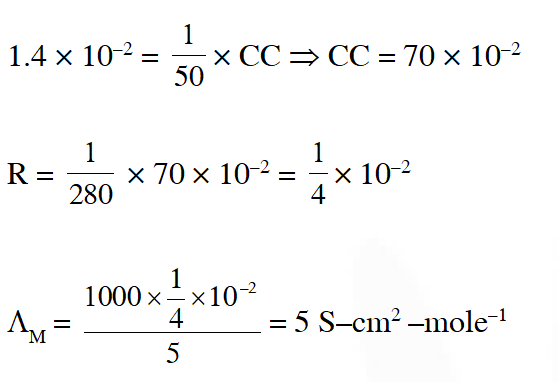Q. The reduction potential of hydrogen half-cell will be negative if :- (1) $\mathrm{p}\left(\mathrm{H}_{2}\right)=2 \operatorname{atm}\left[\mathrm{H}^{+}\right]=1.0 \mathrm{M}$ (2) $\mathrm{p}\left(\mathrm{H}_{2}\right)=2$ atm and $\left[\mathrm{H}^{+}\right]=2.0 \mathrm{M}$ (3) $\mathrm{p}\left(\mathrm{H}_{2}\right)=1$ atm and $\left[\mathrm{H}^{+}\right]=2.0 \mathrm{M}$ (4) $\mathrm{p}\left(\mathrm{H}_{2}\right)=1$ atm and $\left[\mathrm{H}^{+}\right]=1.0 \mathrm{M}$ AIEEE 2011
Ans. (1)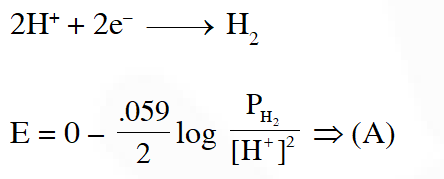Q. The standard reduction potentials for $\mathrm{Zn}^{2+} / \mathrm{Zn}, \mathrm{Ni}^{2+} / \mathrm{Ni}$ and $\mathrm{Fe}^{2+} / \mathrm{Fe}$ are $-0.76,-0.23$ and – 0.44 V respectively. The reaction $\mathrm{X}+\mathrm{Y}^{+2} \rightarrow \mathrm{X}^{2+}+\mathrm{Y}$ will be spontaneous when (1) X = Zn, Y = Ni (2) X = Ni, Y = Fe (3) X = Ni, Y = Zn (4) X = Fe, Y = Zn
Ans. (1) For spontaneous reaction $\mathrm{E}^{\circ}>0 \mathrm{so}(\mathrm{A})$
Q. Given :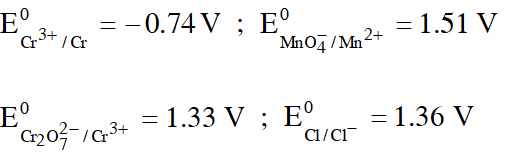Based on the data given above, strongest oxidising agent will be : (1) Cl– (2) $\mathrm{Cr}^{3+}$ (3) $\mathrm{Mn}^{2+}$ (4) $\mathrm{MnO}_{4}^{-}$ JEE-Mains 2013
Ans. (4) Higher the SRP, stronger will be oxidising agent Hence, $\mathrm{MnO}_{4}^{-}$ is stronger oxidising agent.
Q. The equivalent conductance of NaCl at concentration C and at infinite dilution are $\lambda_{\mathrm{C}}$ and $\lambda_{\infty}$ , respectively. The correct relationship between $\lambda_{\mathrm{C}}$ and $\lambda_{\infty}$ is given as: (1) $\lambda_{\mathrm{C}}=\lambda_{\infty}-(\mathrm{B}) \sqrt{\mathrm{C}}$ (2) $\lambda_{\mathrm{C}}=\lambda_{\infty}+(\mathrm{B}) \sqrt{\mathrm{C}}$ (3) $\lambda_{\mathrm{C}}=\lambda_{\infty}+(\mathrm{B}) \mathrm{C}$ (4) $\lambda_{\mathrm{C}}=\lambda_{\infty}-(\mathrm{B}) \mathrm{C}$ JEE-Mains 2014
Ans. (1) Fact
Q. At 298 K, the standard reduction potentials are 1.51 V for $\mathrm{MnO}_{4}-| \mathrm{Mn}^{2+}$ , 1.36 V for $\mathrm{Cl}_{2} | \mathrm{Cl}^{-}$, 1.07 V for $\mathrm{Br}_{2} | \mathrm{Br}^{-},$ and $0.54 \mathrm{V}$ for $\mathrm{I}_{2} | \mathrm{I}^{-} .$ At $\mathrm{pH}=3$, permanganate is expected to oxidize $\left(\frac{\mathrm{RT}}{\mathrm{F}}=0.059 \mathrm{V}\right):-$ (1) $\mathrm{Cl}^{-}$ and $\mathrm{Br}^{-}$ (2) $\mathrm{Cl}^{-}, \mathrm{Br}^{-}$ and $\mathrm{I}^{-}$ (3) $\mathrm{Br}^{-}$ and $\mathrm{I}^{-}$ (4) I- only JEE-Mains (online) 2015
Ans. (3)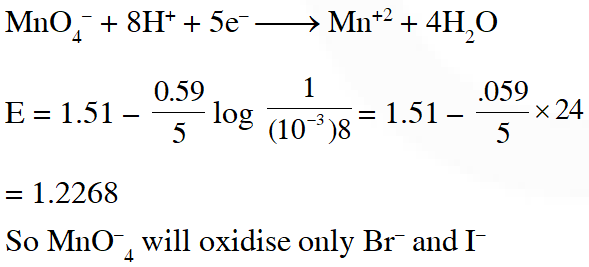Q. A variable, opposite external potential $\left(\mathrm{E}_{\mathrm{ext}}\right)$ is applied to the cell $\mathrm{Zn}\left|\mathrm{Zn}^{2+}(1 \mathrm{M}) \| \mathrm{Cu}^{2+}(1 \mathrm{M})\right| \mathrm{Cu}$, of potential 1.1 V. When $\mathrm{E}_{\mathrm{ext}}<1.1 \mathrm{V}$ and $\mathrm{E}_{\mathrm{ext}}>1.1 \mathrm{V}$ , respectively electrons flow from : (1) anode to cathode in both cases (2) anode to cathode and cathode to anode (3) cathode to anode in both cases (4) cathode to anode and anode to cathode JEE-Mains (online) 2015
Ans. (2) Fact
Q. Two Faraday of electricity is passed through a solution of $\mathrm{CuSO}_{4}$. The mass of copper deposited at the cathode is : (at. mass of Cu = 63.5 amu) (1) 2g         (2) 127 g          (3) 0 g          (4) 63.5 g JEE-Mains 2015
Ans. (4) $2 \mathrm{F}=2 \mathrm{eq}=1$ mole $=63.5 \mathrm{gm}$
Q. Galvanization is applying a coating of :- (1)Zn         (2) Pb         (3) Cr        (4) Cu JEE-Mains 2016
Ans. (1) Galvanization is the process of applying a protective zinc coating of steel or iron, to prevent rusting.
Q. Given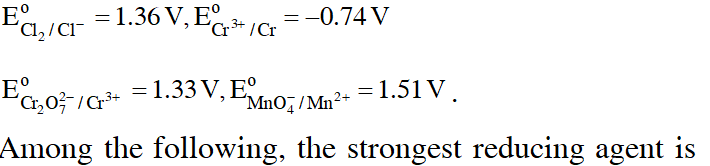(1)Cr (2) $\mathrm{Mn}^{2+}$ (3) $\mathrm{Cr}^{3+}$ (4) $\mathrm{Cl}^{-}$ JEE-Mains 2017
Ans. (1)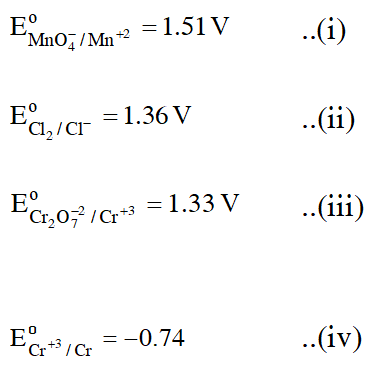Since $\mathrm{Cr}^{+3}$ is having least reducing potential, so Cr is the best Reducing agent.
Q. How long (approximate) should water be electrolysed by passing through 100 amperes current so that the oxygen released can completely burn 27.66 g of diborane ? (Atomic weight of B = 10.8 u) (1)0.8 hours (2) 3.2 hours (3) 1.6 hours (4) 6.4 hours JEE-Mains 2018
Ans. (2)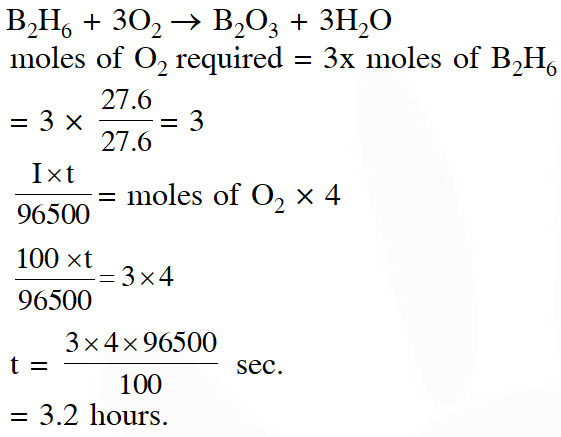Ankit kumar
June 14, 2023, 6:35 a.m.
Plz provide latest question
SAANVI PATNE
June 12, 2021, 11:33 a.m.
Plz provide 2019 and 2020 questions. And more detail solution is required.
Tulasi.U
Jan. 7, 2021, 12:10 p.m.
can u plz post more questions for preparation
harsh
Dec. 30, 2020, 4:25 p.m.
3rd question is full of mistakes.......................................
awatansh sahay
Dec. 18, 2020, 10:28 a.m.
need more questions and more detailed solutions on every problem. questions are good enough
snehith rao
Oct. 10, 2020, 11:34 a.m.
more questions and latest questions
Rajeshwari
Sept. 12, 2020, 2:29 p.m.
Need detailed solutions
Srigowthami
Aug. 30, 2020, 8:03 p.m.
Thanks
pranith
Aug. 28, 2020, 3:58 p.m.
plz make it better
Yashasvi
Aug. 22, 2020, 4:35 p.m.
Sindhuja
Aug. 20, 2020, 8:19 p.m.
Still more questions are required plz add questions of 2019 and 20 questions in it.... And also provide detail solutions
Gautam
Aug. 16, 2020, 10:55 p.m.
Sir , I want to purchase previous years IIT JEE Chapter wise. Chemistry Questions with solution, you have any book for this pl confirm &amp; cost of book
Surya
Aug. 3, 2020, 6:52 p.m.
TRUE IIT ASPIRANT
July 15, 2020, 3:45 p.m.
Plz bro questions are dope but i need clr solutions even i cant solve it
thanu
July 2, 2020, 7:54 p.m.
clear solutions required
L.R.P.R
June 22, 2020, 7:44 a.m.
Clear solutions required
L.R.P
June 22, 2020, 7:44 a.m.
Clear solutions required
Pg
June 21, 2020, 2:32 p.m.
Need more questions for practice
Sai sree
June 18, 2020, 11:29 a.m.
Need some more questions 😎😎😎😎 plzzzzzzzzzzzzzzzzzzzzzzzzzzzzzzzzzzzzzzzzzzzzzzzzzzzzzzzzzzzzzzzzzzzzzzzzzzzzz
Vishnu shedole
May 31, 2020, 3:12 p.m.
Can u provide clear solutions
Akhil
May 22, 2020, 6:55 p.m.
Chill bro
sangamesh A
May 9, 2020, 11:01 p.m.
Excellent
Likhitha .Pasupuleti
March 23, 2020, 6:43 a.m.
Very nice
Likhitha .Pasupuleti
March 23, 2020, 6:42 a.m.
👌👌